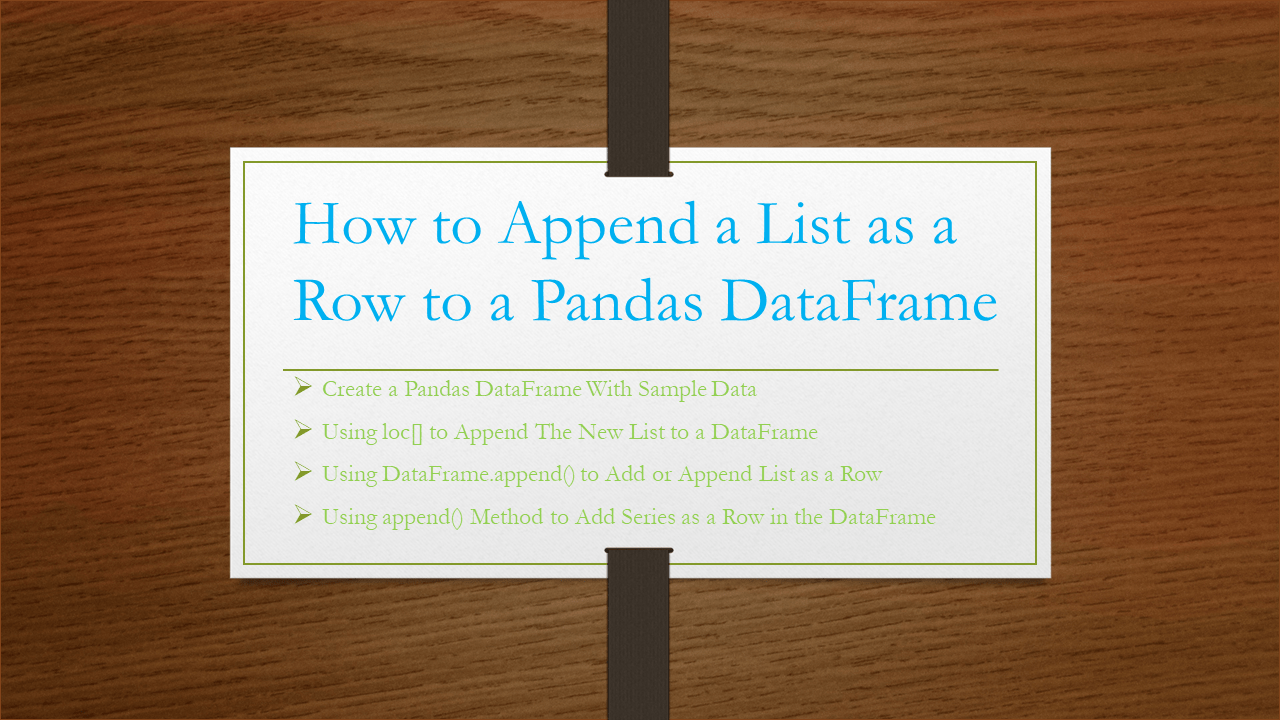# Pandas – Append a List as a Row to DataFrame

pandas support several ways to append a list as a row to DataFrame, In this article, I will explain how to append a python list as a row where ita adds a new rows to the DataFrame with elements specified by a list.

You can use append() method and loc[], iloc[] properties to append/add a list of values as a row in pandas, let’s see these with examples. Use loc vs iloc to understand the differences.

## 1. Quick Examples of pandas append list to DataFrame

Below are some quick examples that aappend list as row to DataFrame.

``````
# New list to append Row to DataFrame
list = ["Hyperion", 27000, "60days", 2000]
df.loc[len(df)] = list

df.iloc = list

# Using append()
list = ["Bigdata", 27000, "40days", 2800]
df2 = df.append(pd.DataFrame([list],
columns=["Courses","Fee","Duration","Discount"]),
ignore_index=True)

# A series object with the same index as DataFrame
df2 = df.append(pd.Series(list, index = ["Courses","Fee","Duration","Discount"]),
ignore_index=True)
``````

Let’s create a DataFrame with a few rows and columns and execute some examples and validate the results. Our DataFrame contains column names `Courses`, `Fee`, `Duration` and `Discount`.

``````
import pandas as pd
technologies= {
'Fee' :[22000,25000,23000,24000,26000],
'Duration':['30days','50days','35days', '40days','55days'],
'Discount':[1000,2300,1000,1200,2500]
}
df = pd.DataFrame(technologies)
print(df)
``````

Yields below output.

``````
Courses    Fee Duration  Discount
0    Spark  22000   30days      1000
1  PySpark  25000   50days      2300
3   Python  24000   40days      1200
4   Pandas  26000   55days      2500
``````

## 2. Using loc[] to Append The New List to a DataFrame

By using `df.loc[index]=list` you can append a list as a row to the DataFrame at a specified Index, In order to add at the end get the index of the last record using `len(df)` function. The below example adds the list `["Hyperion",27000,"60days",2000]` to the end of the pandas DataFrame.

``````
# New list to append Row to DataFrame
list = ["Hyperion", 27000, "60days", 2000]
df.loc[len(df)] = list
print(df)
``````

Yields below output.

``````
Courses    Fee Duration  Discount
0     Spark  22000   30days      1000
1   PySpark  25000   50days      2300
3    Python  24000   40days      1200
4    Pandas  26000   55days      2500
5  Hyperion  27000   60days      2000
``````

Use `df.iloc=list` to append the row to the second position of the DataFrame as Index starts from zero.

``````
# New list to append DataFrame
list = ["Oracle", 20000, "60days", 2000]
# using iloc[] method
df.iloc = list
print(df)
``````

Yields below output.

``````
Courses    Fee Duration  Discount
0   Spark  22000   30days      1000
1  Oracle  20000   60days      2000
3  Python  24000   40days      1200
4  Pandas  26000   55days      2500
``````

## 3. Using DataFrame.append() to Add or Append List as a Row

Alternatively, you can also use DataFrame.append() function to add a row to the DataFrame. This by default adds a row at the end. You can Get Column Names as a List From Pandas DataFrame and use it in this example.

``````
# New list for append into DataFrame
list = ["Bigdata", 27000, "40days", 2800]
# Using append to add the list to DataFrame
df2 = df.append(pd.DataFrame([list], columns=["Courses","Fee","Duration","Discount"]), ignore_index=True)
print(df2)
``````

Yields below output.

``````
Courses    Fee Duration  Discount
0    Spark  22000   30days      1000
1  PySpark  25000   50days      2300
3   Python  24000   40days      1200
4   Pandas  26000   55days      2500
5  Bigdata  27000   40days      2800
``````

## 4. Append Series as a Row to the DataFrame

Using append() you can also append series as a row to the DataFrame.

``````
# New list for append into DataFrame
list = ["Bigdata", 27000, "40days", 2800]
# A series object with the same index as DataFrame
df2 = df.append(pd.Series(list, index = ["Courses","Fee","Duration","Discount"]), ignore_index=True)
print(df2)
``````

Yields below output.

``````
Courses    Fee Duration  Discount
0    Spark  22000   30days      1000
1  PySpark  25000   50days      2300
3   Python  24000   40days      1200
4   Pandas  26000   55days      2500
5  Bigdata  27000   40days      2800
``````

### Conclusion

In this article, you have learned how to append a list as a row to Pandas DataFrame using `DataFrame.loc[]`, `DataFrame.iloc[]`, `DataFrame.append()` methods. Using these you can append a row at any position/index.

Happy Learning !!

### NNK

SparkByExamples.com is a Big Data and Spark examples community page, all examples are simple and easy to understand and well tested in our development environment Read more ..